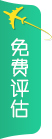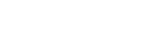• *
• *
• *

意向国家

• *

攻读学位

• *

所在地区我接受并同意 《用户服务条款》《隐私权相关政策》
【前途首页】 新东方前途出国留学，您理想的留学伙伴！
English Wibsite| 微信xdfqiantu

| 各地分公司

|关于前途
4分制？4.3分制？12分制？GPA到底怎么算？分类：

GPA是学校判断申请者是否具有扎实专业知识以及潜在学术能力的基础，是体现申请者学习能力的一个可量化的指标。

(70*4+80*4+100*2)/(4+4+2)=80

「北大算法」
90=4.0，85=3.7，82=3.3，78=3.0，75=2.7，72=2.3，69=2.0，66=1.7，63=1.3，60=1.0，<60=0.0

「浙大算法」
85～100=4.0，60～84=1.5～3.9（1分为0.1）

「WES算法」

A=4.0；A-=3.67；B+=3.33；B=3.0；B-=2.67；C+=2.33；C=2.00；C-=1.67；D+=1.33；D=1.00；D-=0.67；F=0

GPA就是将每门课的学分乘以其对应的换算后绩点，所有乘积加和之后再除以总学分数，就是GPA了。

» 美国

①美国GPA换算

GPA成绩其实是由你所在的学校和你要申请的学校决定的，不能笼统概括。

②美国留学GPA要求

3.7+：基本上可以申请TOP30的学校；

3.5+：可以尝试去申请TOP50的学校，如果软实力背景较强，可以试着冲刺TOP30的学校；

3.0+：可以申请TOP100以内的学校，多提升软实力以弥补GPA上的缺陷，也可以冲刺TOP50的学校；

3.0-：申请TOP100的美国大学可能较为困难，很大几率在网申时就会被刷掉。

GPA虽然看起来只是一个简单的数字，但却很直观的将你的学业情况呈现出来，同时反应出了真实的学习能力。

###### 4分制？4.3分制？12分制？GPA到底怎么算？GPA是学校判断申请者是否具有扎实专业知识以及潜在学术能力的基础，是体现申请者学习能力的一个可量化的指标。

(70*4+80*4+100*2)/(4+4+2)=80

「北大算法」
90=4.0，85=3.7，82=3.3，78=3.0，75=2.7，72=2.3，69=2.0，66=1.7，63=1.3，60=1.0，<60=0.0

「浙大算法」
85～100=4.0，60～84=1.5～3.9（1分为0.1）

「WES算法」

A=4.0；A-=3.67；B+=3.33；B=3.0；B-=2.67；C+=2.33；C=2.00；C-=1.67；D+=1.33；D=1.00；D-=0.67；F=0

GPA就是将每门课的学分乘以其对应的换算后绩点，所有乘积加和之后再除以总学分数，就是GPA了。

» 美国

①美国GPA换算

GPA成绩其实是由你所在的学校和你要申请的学校决定的，不能笼统概括。

②美国留学GPA要求

3.7+：基本上可以申请TOP30的学校；

3.5+：可以尝试去申请TOP50的学校，如果软实力背景较强，可以试着冲刺TOP30的学校；

3.0+：可以申请TOP100以内的学校，多提升软实力以弥补GPA上的缺陷，也可以冲刺TOP50的学校；

3.0-：申请TOP100的美国大学可能较为困难，很大几率在网申时就会被刷掉。

GPA虽然看起来只是一个简单的数字，但却很直观的将你的学业情况呈现出来，同时反应出了真实的学习能力。

• 您的姓名：
• 手机号码：